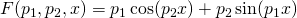## Simple nonlinear least squares curve fitting in Python

December 6th, 2013 | Categories: math software, programming, python | Tags:

A question I get asked a lot is ‘How can I do nonlinear least squares curve fitting in X?’ where X might be MATLAB, Mathematica or a whole host of alternatives.  Since this is such a common query, I thought I’d write up how to do it for a very simple problem in several systems that I’m interested in

This is the Python version. For other versions,see the list below

The problem

xdata = -2,-1.64,-1.33,-0.7,0,0.45,1.2,1.64,2.32,2.9
ydata = 0.699369,0.700462,0.695354,1.03905,1.97389,2.41143,1.91091,0.919576,-0.730975,-1.42001

and you’d like to fit the functionusing nonlinear least squares.  You’re starting guesses for the parameters are p1=1 and P2=0.2

For now, we are primarily interested in the following results:

• The fit parameters
• Sum of squared residuals

Future updates of these posts will show how to get other results such as confidence intervals. Let me know what you are most interested in.

Python solution using scipy

Here, I use the curve_fit function from scipy

import numpy as np
from scipy.optimize import curve_fit

xdata = np.array([-2,-1.64,-1.33,-0.7,0,0.45,1.2,1.64,2.32,2.9])
ydata = np.array([0.699369,0.700462,0.695354,1.03905,1.97389,2.41143,1.91091,0.919576,-0.730975,-1.42001])

def func(x, p1,p2):
return p1*np.cos(p2*x) + p2*np.sin(p1*x)

popt, pcov = curve_fit(func, xdata, ydata,p0=(1.0,0.2))

The variable popt contains the fit parameters

array([ 1.88184732,  0.70022901])

We need to do a little more work to get the sum of squared residuals

p1 = popt
p2 = popt
residuals = ydata - func(xdata,p1,p2)
fres = sum(residuals**2)

which gives

0.053812696547933969
1. Thanks a lot for the clear information and examples. I have a question you could probably shed some light on.Since I started my Ph. D. I decided to use python (numpy,scipy,etc) as my main scientific software tool. So far I am very pleased with the decision I made, however, now I am trying to solve a nonlinear optimisation problem which basically consist in fitting some data to a cascade of linear filtera and some static nonlinearities. I put together a script in python which uses “scipy.optimize.leastsq()”. I haven’t been able to get an acceptable fit so far and the speed is not great either. So the question is in your experience would you say that is a good option to use this function, or the matlab ones are better quality? and in your mind which matlab function would be equivalent to this python one?

2. Hi Carlos

I’ve never done a speed/quality comparison between these optimisation functions on different systems I’m afraid. All I can say is that I’ve not had a problem with the Python ones so far.

Best Wishes,
Mike

3. Hello Mike,

Thanks for your promptly answer.Perhaps in the near future I will carry out some comparison tests.If I get any significant/interesting results I will share them here.

Cheers,
Carlos.

4. Hi,

you can use the full_output flag (borrowed from the scipy.optimize.leastsq function) to obtain further information about the solution:

popt, pcov, infodict, mesg, ier = curve_fit(func, xdata, ydata,p0=(1.0,0.2),full_output=1)

With that call you can now estimate the residuals as:

fres = sum(infodict[‘fvec’]**2)

BTW, great posts.
Paulo Xavier Candeias

5. Thanks, Paulo.

6. Hi again,

@Paulo Thanks for the tip.With this information relevant postprocessing ca be achieved.

Well after a reading from internet,SciPy and matlab docs and trying out some ideas my conclusion regarding the performance for nonlinear regression goes as follows:

Python: Using scipy.optimize methods, either leastsq or curve_fit, is a working way to
get a solotion for a nonlinear regression problem.As I understood the solver
is a wrapper to the MINPACK fortran library, at least in the case of the L-M
algorithm, which is the one I am working with.This suppose to avoid wheel
reinventing stages.However in my case this had two downsides.First,passing a
python numpy.array of dimension other than (dimesnion,)
seems not to work properly. And second, I wanted to use a slightly modification
of the classical L-M algorithm but since the code is not in python then it was
not very easy to do this.
Matlab: Mike kindly explained different ways to get a nonlinear optimisation problem
solved using no toolbox, stat,opt TBs or using the NAG library.I have
just looked into the ‘nlinfit’ from the stats TB. Comparing this against the
scipy alternatives I can say that the main difference algoritmic-wise is that
this routine incorporates some enhancements to the classical algorithmm.These
include iterative re-weighting and robust strategies.

I summary I would say that if using scipy routines is enough for your case then there is
not need to make things more complex. However, if you ever find yourself struggling to get
the desired performance it might be worth looking into matlab or other software tool which implements some improvements to the basic algorithm.Or try to implement any enhancement you might need/want to try in python.This can be time consuming and error prone though.Anyway, I hope this can be useful for other people.

Carlos.

7. Thank you very much for the most clear information on how to work with curve_fit. I have searched pretty much everywhere in Google and this explanation seems to be the easiest to follow. Wonder why scipy docs don’t have examples like these?!!

S.Srivatsan

8. Hi Mike,

I found this example very useful for my quick prototyping. Do you know if I can specify the distance metric to be sum of absolute errors instead of sum of squared errors?

Anurag

9. Hi Anurag,

I think not, the problem resides in the mathematical formulation of the problem. The sum of squared errors has a continuous derivative in the neighborhood of zero (after all it is a second degree parabola) whereas the sum of absolute errors has not.

Paulo Xavier Candeias

10. Hello Mike,

When I use scipy.optimize.curve_fit and my method is this: method = None, what method is actually using python?
regards